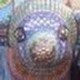# The Unapologetic Mathematician

## The Character Table of S4

Let’s use our inner tensor products to fill in the character table of$S_4$. We start by listing out the conjugacy classes along with their sizes:$\displaystyle\begin{array}{cc}e&1\\(1\,2)&6\\(1\,2)(3\,4)&3\\(1\,2\,3)&8\\(1\,2\,3\,4)&6\end{array}$

Now we have the same three representations as in the character table of$S_3$: the trivial, the signum, and the complement of the signum in the defining representation. Let’s write what we have.$\displaystyle\begin{array}{c|ccccc}&e&(1\,2)&(1\,2)(3\,4)&(1\,2\,3)&(1\,2\,3\,4)\\\hline\chi^\mathrm{triv}&1&1&1&1&1\\\mathrm{sgn}&1&-1&1&1&-1\\\chi^\perp&3&1&-1&0&-1\\\vdots&\vdots&\vdots&\vdots&\vdots&\vdots\end{array}$

Just to check, we calculate$\displaystyle\langle\chi^\perp,\chi^\perp\rangle=(1\cdot3\cdot3+6\cdot1\cdot1+3\cdot-1\cdot-1+8\cdot0\cdot0+6\cdot-1\cdot-1)/24=1$

so again,$\chi^\perp$ is irreducible.

But now we can calculate the inner tensor product of$\mathrm{sgn}$ and$\chi^\perp$. This gives us a new line in the character table:$\displaystyle\begin{array}{c|ccccc}&e&(1\,2)&(1\,2)(3\,4)&(1\,2\,3)&(1\,2\,3\,4)\\\hline\chi^\mathrm{triv}&1&1&1&1&1\\\mathrm{sgn}&1&-1&1&1&-1\\\chi^\perp&3&1&-1&0&-1\\\mathrm{sgn}\otimes\chi^\perp&3&-1&-1&0&1\\\vdots&\vdots&\vdots&\vdots&\vdots&\vdots\end{array}$

which we can easily check to be irreducible.

Next, we can form the tensor product$\chi^\perp\otimes\chi^\perp$, which has values\displaystyle\begin{aligned}\chi^\perp\otimes\chi^\perp\left(e\right)&=9\\\chi^\perp\otimes\chi^\perp\left((1\,2)\right)&=1\\\chi^\perp\otimes\chi^\perp\left((1\,2)(3\,4)\right)&=1\\\chi^\perp\otimes\chi^\perp\left((1\,2\,3)\right)&=0\\\chi^\perp\otimes\chi^\perp\left((1\,2\,3\,4)\right)&=1\end{aligned}

Now, this isn’t irreducible, but we can calculate inner products with the existing irreducible characters and decompose it as$\displaystyle\chi^\perp\otimes\chi^\perp=\chi^\mathrm{triv}+\chi^\perp+\mathrm{sgn}\otimes\chi^\perp+\chi^{(5)}$

where$\chi^{(5)}$ is what’s left after subtracting the other three characters. This gives us one more line in the character table:$\displaystyle\begin{array}{c|ccccc}&e&(1\,2)&(1\,2)(3\,4)&(1\,2\,3)&(1\,2\,3\,4)\\\hline\chi^\mathrm{triv}&1&1&1&1&1\\\mathrm{sgn}&1&-1&1&1&-1\\\chi^\perp&3&1&-1&0&-1\\\mathrm{sgn}\otimes\chi^\perp&3&-1&-1&0&1\\\chi^{(5)}&2&0&2&-1&0\end{array}$

and we check that$\displaystyle\langle\chi^{(5)},\chi^{(5)}\rangle=(1\cdot2\cdot2+6\cdot0\cdot0+3\cdot2\cdot2+8\cdot-1\cdot-1+6\cdot0\cdot0)/24=1$

so$\chi^{(5)}$ is irreducible as well.

Now, we haven’t actually exhibited these representations explicitly, but there is no obstacle to carrying out the usual calculations. Matrix representations for$V^\mathrm{triv}$ and$V^\mathrm{sgn}$ are obvious. A matrix representation for$V^\perp$ comes just as in the case of$S_3$ by finding a basis for the defining representation that separates out the copy of$V^\mathrm{triv}$ inside it. Finally, we can calculate the Kronecker product of these matrices with themselves to get a representation corresponding to$\chi^\perp\otimes\chi^\perp$, and then find a basis that allows us to split off copies of$V^\mathrm{triv}$,$V^\perp$, and$V^\mathrm{sgn}\otimes V^\perp$.

November 8, 2010 -

1. Is there a general procedure for filling out the character table, or is it a matter of trying different combinations until you find a new irreducible character orthogonal to the ones you’ve already got (and iterating until you have a full basis)?Comment by Joe English | November 9, 2010 | Reply

2. We’ll come up with a general straightforward position for symmetric groups eventually.Comment by John Armstrong | November 9, 2010 | Reply

3. […] We can check this in the case of and , since we have complete character tables for both of them: […]

Pingback by Decomposing the Left Regular Representation « The Unapologetic Mathematician | November 17, 2010 | Reply

4. Small error, it’s the complement of the trivial rep, not the signum….Comment by Charles Waldman | May 2, 2013 | Reply